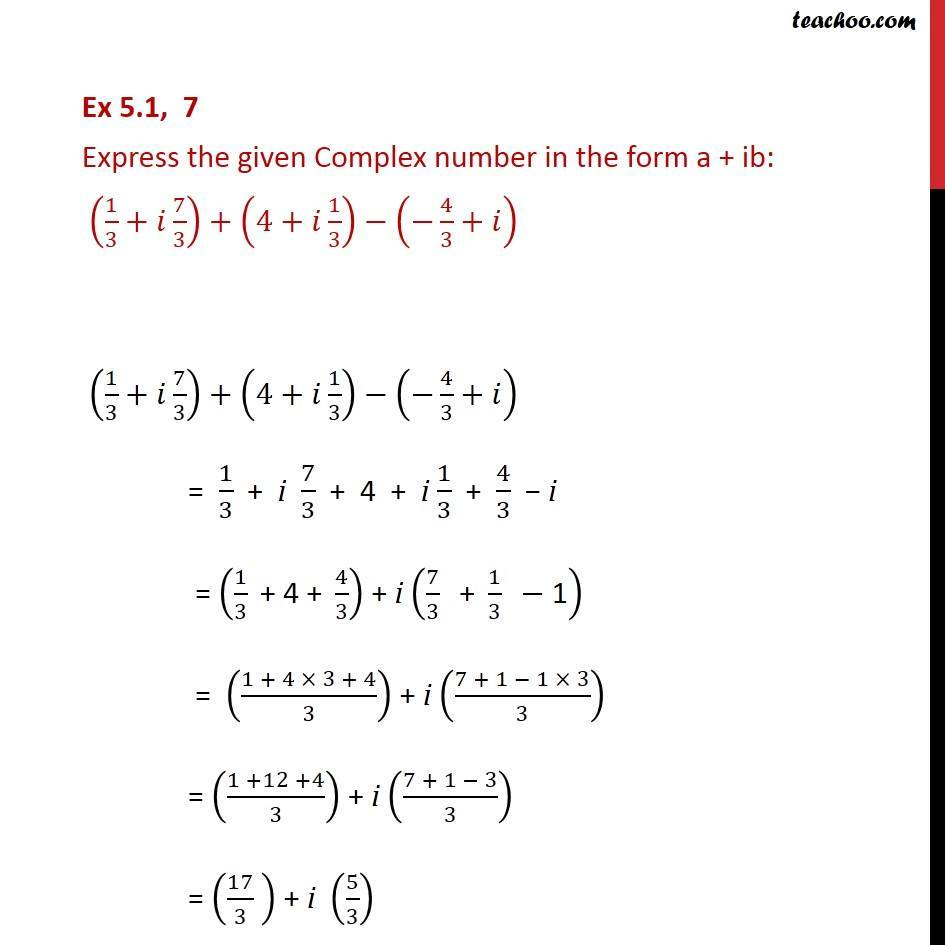Convert to a + ib form

Chapter 4 Class 11 Complex Numbers
Concept wiseLearn in your speed, with individual attention - Teachoo Maths 1-on-1 Class

### Transcript

Ex 4.1, 7 Express the given Complex number in the form a + ib: (1/3+ 7/3)+(4+ 1/3) ( 4/3+ ) (1/3+ 7/3)+(4+ 1/3) ( 4/3+ ) = 1/3 + i 7/3 + 4 + i 1/3 + 4/3 i = (1/3 " + 4 + " 4/3) + i (7/3 " + " 1/3 " " "1" ) = ((1 + 4 3 + 4)/3) + i ((7 + 1 1 3)/3) = ((1 +12 +4)/3) + i ((7 + 1 3)/3) = (17/3 ) + i (5/3)